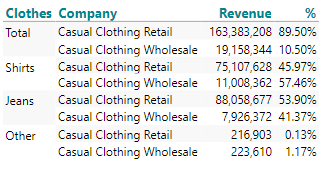# Calculations with specific level

Hi all,

I have the following table in TARGIT (Column A-C), but need to realize the calculation in Column D and E.

All dimensions are user dimensions.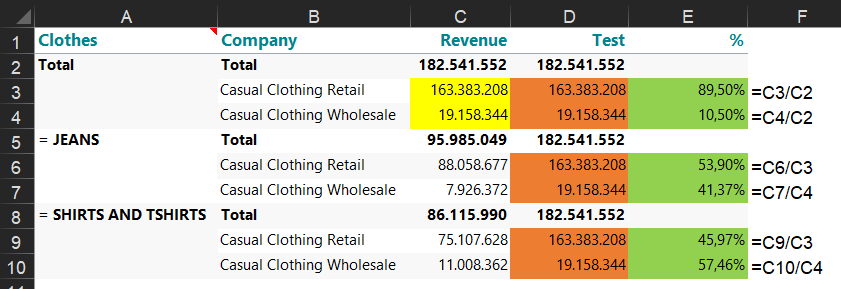I tried a calculated measure sum(0, d1(l(1,1)), m1) to reference always to the first rows, but this seems to be not the answer.

Does anybody have an idea? Thank you very much!

• Hi Marlene

Just a quick suggestion: Maybe you could make a new column that counts 0, 1, 2, 0, 1, 2 etc. with syntax mod(xx,3). And then the syntax for column D could be: if the new coulmn = 0 then take the first row, else if new column = 1 then take the second row, else take then third row.

But of course it only works if the number of companies in column B is always the same :-)

• Hi Marlene

I've come up with a solution that requires a few intermediate calculations.

The solution below can be "compressed" to fewer intermediates, but to understand what's going on, I have done it step by step.

First calculation is a count of siblings: sum(d1,d1:0(s),m1)

From now on this will be the m2 measure.

This will result in a count within each group like this: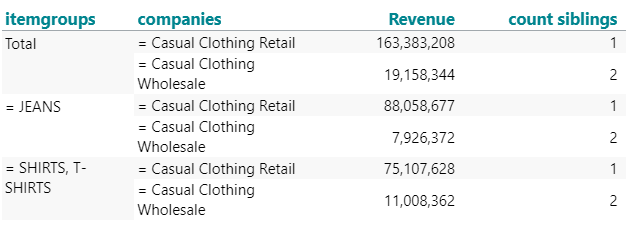Next step is to locate where a group ends - this is a prerequisite to identify the first group:

if sum(d1, 0, m2) < sum(d1, 1, m2, 0) then 0 else 1

This will check if the next value is bigger (which would mean that we are still in the same group) and set a flag each time a group ends.

From now on this is the m3 measure.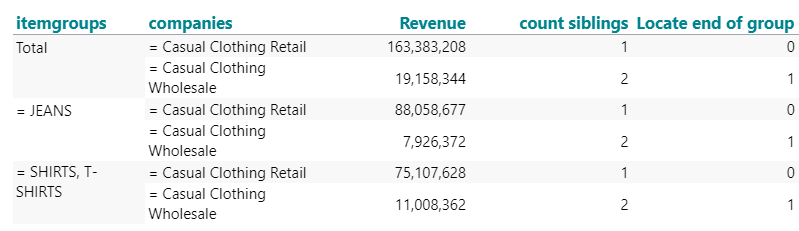With these 2 calculations, we now have the building blocks to identify the 1st group and transfer these values with this syntax:

if sum(d1, -1:-100, m3, 0) = 0 then sum(d1, all(s), m1) else 0

We are checking on the flags from the previous calculation and as long as the sum of all the previous rows is 0, we haven't met the 1st flag yet and we can safely sum up siblings since this will be siblings from the first group. Once all the previous flags add up to more than zero, we are past the first flag.

This will now be measure m4.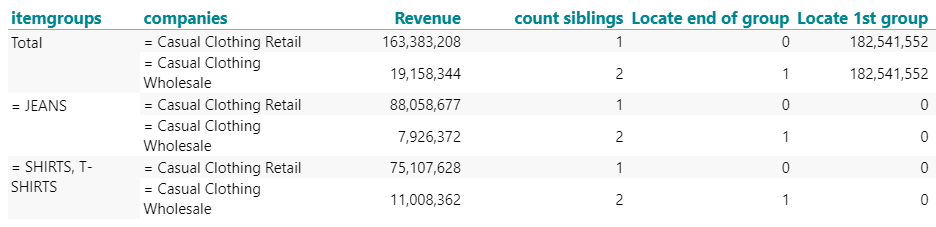Now we will transfer the company values from the first group to the same company in each group. Since there is no way to loop through values, we are going to have to do a nested if sentence (I'm hoping you don't have an infinite number of companies).

Example syntax:

if sum(d1, 0, m2) = 1 then sum(d1, d1, m1) else
if sum(d1, 0, m2) = 2 then sum(d1, d2, m1) else
if sum(d1, 0, m2) = 3 then sum(d1, d3, m1) else
if sum(d1, 0, m2) = 4 then sum(d1, d4, m1) else
if sum(d1, 0, m2) = 5 then sum(d1, d5, m1) else 0

This will be measure m5 from now on.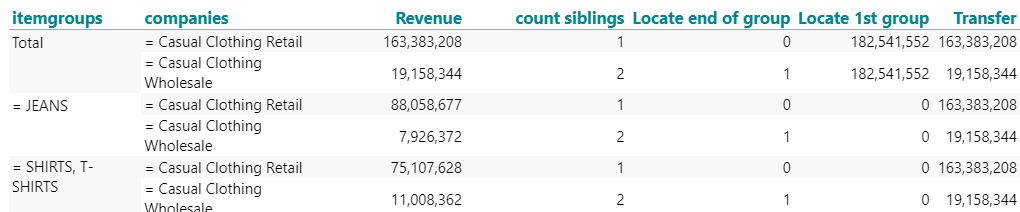We are almost there :-)
Now a simple if sentence to merge the 2 last calculations and create a measure which holds the correct numbers for your final percentage calculation.

if sum(d1, 0, m4) > 0 then sum(d1, 0, m4) else sum(d1, 0, m5)

This will be measure m6.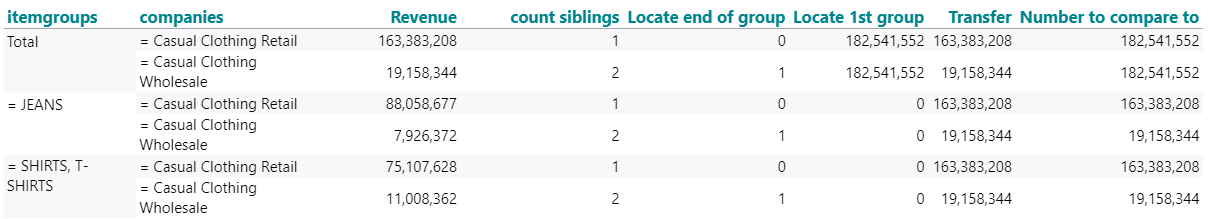And the the icing on the cake (while hiding all the prerequisites).

sum(d1, 0, m1) / sum(d1, 0, m6)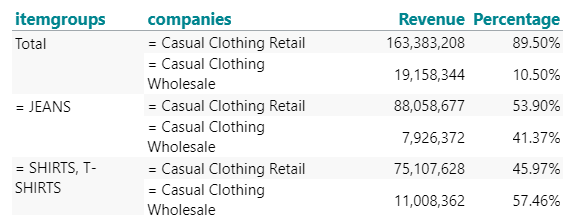Is this a solution that can help you?

• Hi and sorry for the late reply, I was on vacation. The solution works really good in my test. Now Jens Schomacker can use this in the live environment :-)